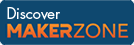Cody

# Problem 1997. Compute Fibonacci Number

Created by Alexander in Community

Compute the n-th Fibonacci Number

f(0) = 0, f(1) = 1, f(2) = 1, f(3) = 2, ... f(42) = 267914296

### Solution Stats

46.57% Correct | 53.43% Incorrect
Last solution submitted on Mar 12, 2019

#### TagsMATLAB and Simulink resources for Arduino, LEGO, and Raspberry Pi